# Arrow functions in Javascript

Arrow Functions Javascript

Now, when we know much about the concept of functions, let’s now concentrate on something called arrow functions. These are the functions that make the syntax of functions even smaller. They do not have any name and we do not need any function keyword to declare the function(what we have been doing for a long). But remember that this thing is limited, and cannot be used in all situations.

Below is the syntax for the arrow functions. You can understand the arrow function with help of the following example –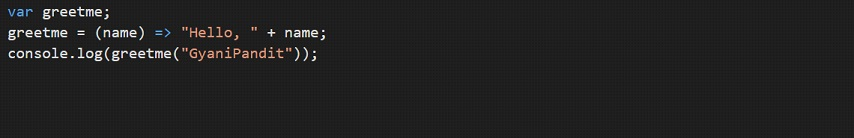As shown in the above method, this is how we can use the arrow functions. Let’s have a look at the output now –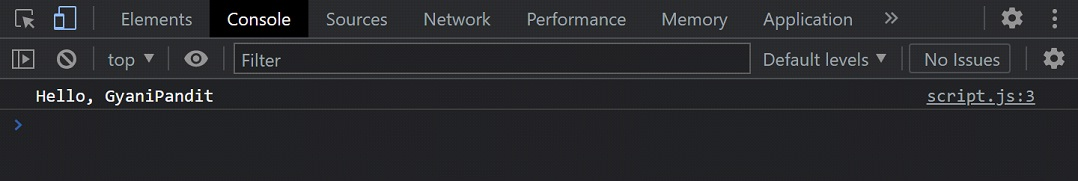As you can see, we could make use of the arrow functions. Well, when we are having only one statement in our arrow function, then they return a value by default, like the above one. Here, we are executing the arrow function, and find that it returns that string.

So, even if you do not write the return keyword, the value is going to be returned. But if the arrow function is having multiple lines, then we are supposed to make use of the return keyword.

Have a look at the below program, where we are having multiple statements in the arrow function. In the function below, we are calculating the power of one number over another number, and then returning the result.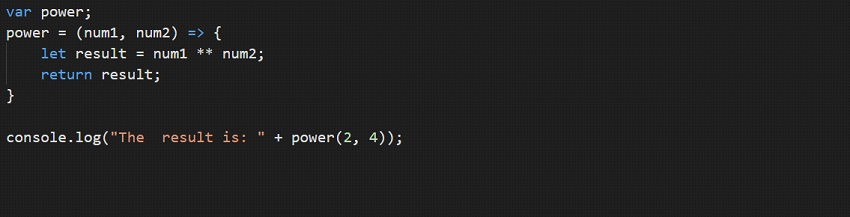As you can see, we had to specify the return keyword along with the value to return. This way, we can make use of the arrow functions, in our programs.

Also, if the arrow function is not going to have any arguments, you should give empty brackets there.

Have a look at the below program, where we are not giving any parameters., let’s have a look at the output –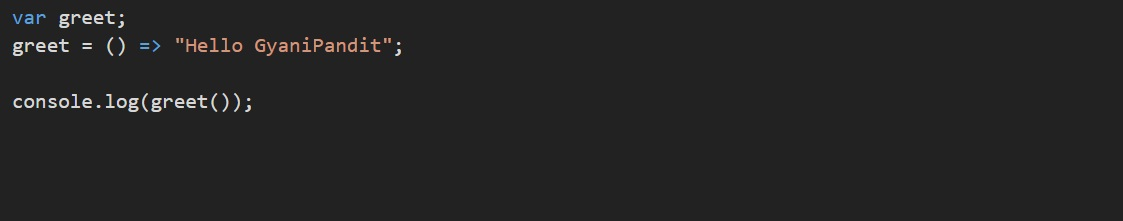Now, let’s have a look at the output for the above-given code-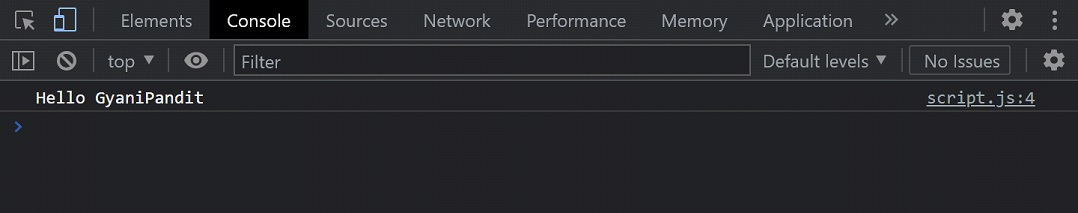As you can see, we have taken several examples for understanding the arrow functions. Ultimately, these are some functions, and the syntax we can say belongs to the simple category. All we are doing is creating a variable, and then some parameters into the brackets, and then an arrow(like this =>), and then we are writing our function code. At times, when we would need the arrow functions, we would make use of them. You can also try out some examples to get more familiar with the concept.